# Obstacles

Using the predefined obstacle templates, a variety of obstacle types can be created which are superimposed on the existing soft-soil road geometry.

## Implementation

In order to properly position the obstacle, the X and Y coordinate of the obstacle’s center as well as the THETA angle, which defines its orientation, must be provided, as shown in Fig. 1.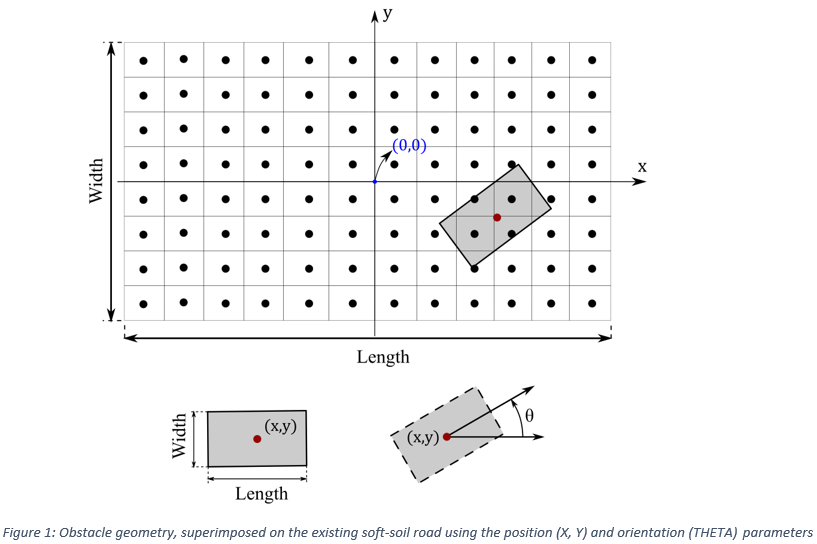By default, each obstacle is created as deformable, making use of the global soft-soil parameters (see Fig. 2). In order to change its default state, the optional parameter MAT can be set to ‘RIGID’ or a user-defined ‘MAT_LABEL’. More specifically, in the first case, the obstacle is rigid (non-deformable) and the tire-rigid road interaction is implemented using the Fiala tire model (see Fig. 3). On the other hand, in the second case the obstacle is still deformable. However, instead of the global soft-soil parameters, a new set of parameters is used for the obstacle’s region which is defined in the new block ‘MAT_LABEL’, as depicted in Fig. 4. Finally, if the block ‘MAT_LABEL’ cannot be found in the road data (.rdf) file, the simulation proceeds using the global soft-soil parameters.

$-----------------------------------------------------SOIL_PROPERTIES [PROPERTIES] PHI = 0.649$ Angle of internal shearing resistance [angle]
C                    = 800.0      $Soil apparent cohesion [force/length**2] KX0 = 0.043$ Shear deformation module [length/angle]
KX1                  = 0.036      $Shear deformation module [length] KY0 = 0.020$ Shear deformation module [length/angle]
KY1                  = 0.013      $Shear deformation module [length] KC = 1370.0$ Pressure-sinkage parameter [force/length**(n+1)]
KPHI                 = 8.14E5     $Pressure-sinkage parameter [force/length**(n+2)] SINKAGE_EXPONENT = 1.0$ Sinkage exponent n [-]
C1                   = 0.4        $Parameter for wheel angle of maximum normal stress [-] C2 = 0.15$ Parameter for wheel angle of maximum normal stress [-]
SOIL_STIFFNESS       = 8.14E6     $Soil elastic stiffness [force/length**3] SOIL_DAMPING = 500.0$ Soil damping [force*time/length]
SOIL_DENSITY         = 1600.0     $Soil density [mass/length**3]$--------------------------------------------------------------RECTANGULAR_OBSTACLE
[RECT_OBSTACLE]
X                    = 0.0        $X coordinate of obstacle's center Y = 0.0$ Y coordinate of obstacle's center
Z                    = 0.0        $Z coordinate of obstacle LENGTH = 1.0$ Length of obstacle
WIDTH                = 0.5        $Width of obstacle THETA = 0.0$ Theta (orientation) angle of obstacle with respect to road z-axis
UMIN                 = 0.8        $Parameter for tire-rigid road interaction (Fiala tire model) UMAX = 1.0$ Parameter for tire-rigid road interaction (Fiala tire model)
CSLIP                = 20000.0    $Parameter for tire-rigid road interaction (Fiala tire model) CALPHA = 44000.0$ Parameter for tire-rigid road interaction (Fiala tire model)

Figure 2: Example of deformable rectangular obstacle, using the global soft-soil parameters

$-----------------------------------------------------RECTANGULAR_OBSTACLE [RECT_OBSTACLE] MAT = 'RIGID'$ Obstacle's material
X                    = 0.0        $X coordinate of obstacle's center Y = 0.0$ Y coordinate of obstacle's center
Z                    = 0.0        $Z coordinate of obstacle LENGTH = 1.0$ Length of obstacle
WIDTH                = 0.5        $Width of obstacle THETA = 0.0$ Theta (orientation) angle of obstacle with respect to road z-axis
UMIN                 = 0.8        $Parameter for tire-rigid road interaction (Fiala tire model) UMAX = 1.0$ Parameter for tire-rigid road interaction (Fiala tire model)
CSLIP                = 20000.0    $Parameter for tire-rigid road interaction (Fiala tire model) CALPHA = 44000.0$ Parameter for tire-rigid road interaction (Fiala tire model)

Figure 3: Example of rigid rectangular obstacle

$-----------------------------------------------------RECTANGULAR_OBSTACLE [RECT_OBSTACLE] MAT = 'MAT1'$ Obstacle's material
X                    = 0.0        $X coordinate of obstacle's center Y = 0.0$ Y coordinate of obstacle's center
Z                    = 0.0        $Z coordinate of obstacle LENGTH = 1.0$ Length of obstacle
WIDTH                = 0.5        $Width of obstacle THETA = 0.0$ Theta (orientation) angle of obstacle with respect to road z-axis
UMIN                 = 0.8        $Parameter for tire-rigid road interaction (Fiala tire model) UMAX = 1.0$ Parameter for tire-rigid road interaction (Fiala tire model)
CSLIP                = 20000.0    $Parameter for tire-rigid road interaction (Fiala tire model) CALPHA = 44000.0$ Parameter for tire-rigid road interaction (Fiala tire model)
$-----------------------------------------------------MATERIAL1 [MAT1] MU = 1.0$ Friction coefficient
PHI                  = 0.649      $Angle of internal shearing resistance [angle] C = 800.0$ Soil apparent cohesion [force/length**2]
KX0                  = 0.043      $Shear deformation module [length/angle] KX1 = 0.036$ Shear deformation module [length]
KY0                  = 0.020      $Shear deformation module [length/angle] KY1 = 0.013$ Shear deformation module [length]
KC                   = 1370.0     $Pressure-sinkage parameter [force/length**(n+1)] KPHI = 7.14E5$ Pressure-sinkage parameter [force/length**(n+2)]
SINKAGE_EXPONENT     = 1.0        $Sinkage exponent n [-] C1 = 0.4$ Parameter for wheel angle of maximum normal stresss [-]
C2                   = 0.15       $Parameter for wheel angle of maximum normal stresss [-] SOIL_STIFFNESS = 7.14E6$ Soil elastic stiffness [force/length**3]
SOIL_DAMPING         = 500.0      $Soil damping [force*time/length] SOIL_DENSITY = 1600.0$ Soil density [mass/length**3]

Figure 4: Example of deformable rectangular obstacle, using the soft-soil parameters defined in 'MAT1' block

### Obstacle types

In the current tire model, the following obstacle types can be used:
• Rectangular obstacle
• Circular obstacle
• Bump obstacle
• Ramp obstacle
• Roof obstacle
• Sine obstacle
• Sine-sweep obstacle (Linear/Logarithmic)
• Plank obstacle (Beveled edges/Round edges)
• Custom obstacle

## Rectangular Obstacle

To create a rectangular obstacle, a new block (RECT_OBSTACLE block) is necessary in the road data (.rdf) file that contains the following parameters: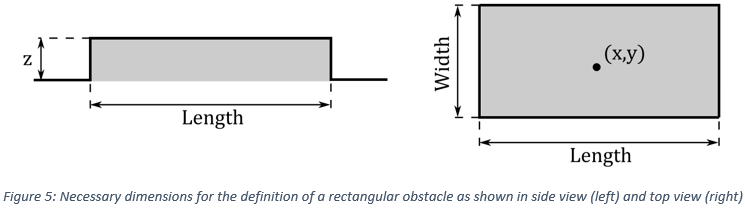Parameter Description Units
X X coordinate of obstacle’s center Length
Y Y coordinate of obstacle’s center Length
Z Z coordinate of obstacle Length
LENGTH Length of obstacle Length
WIDTH Width of obstacle Length
THETA Theta (orientation) angle of obstacle Angle
UMIN Parameter for tire-rigid road interaction (Fiala tire model) -
UMAX Parameter for tire-rigid road interaction (Fiala tire model) -
CSLIP Parameter for tire-rigid road interaction (Fiala tire model) Force
CALPHA Parameter for tire-rigid road interaction (Fiala tire model) Force
MAT Obstacle’s material. Optional parameter, default value is ‘SOFT_SOIL’ -

## Circular Obstacle

To create a circular obstacle, a new block (CIRC_OBSTACLE block) is necessary in the road data (.rdf) file that contains the following parameters: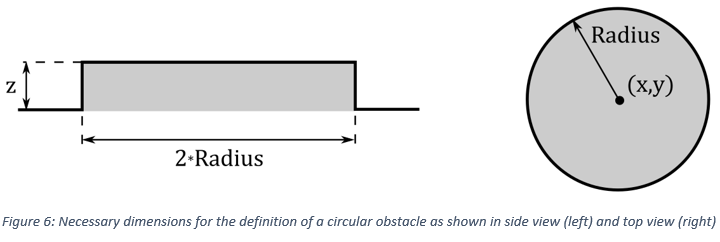Parameter Description Units
X X coordinate of obstacle’s center Length
Y Y coordinate of obstacle’s center Length
Z Z coordinate of obstacle Length
UMIN Parameter for tire-rigid road interaction (Fiala tire model) -
UMAX Parameter for tire-rigid road interaction (Fiala tire model) -
CSLIP Parameter for tire-rigid road interaction (Fiala tire model) Force
CALPHA Parameter for tire-rigid road interaction (Fiala tire model) Force
MAT Obstacle’s material. Optional parameter, default value is ‘SOFT_SOIL’ -

## Bump Obstacle

To create a bump obstacle, a new block (BUMP_OBSTACLE block) is necessary in the road data (.rdf) file that contains the following parameters: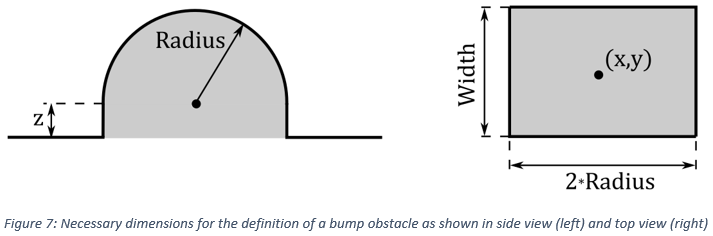Parameter Description Units
X X coordinate of obstacle’s center Length
Y Y coordinate of obstacle’s center Length
Z Z coordinate of bump's center Length
WIDTH Width of obstacle Length
THETA Theta (orientation) angle of obstacle Angle
UMIN Parameter for tire-rigid road interaction (Fiala tire model) -
UMAX Parameter for tire-rigid road interaction (Fiala tire model) -
CSLIP Parameter for tire-rigid road interaction (Fiala tire model) Force
CALPHA Parameter for tire-rigid road interaction (Fiala tire model) Force
MAT Obstacle’s material. Optional parameter, default value is ‘SOFT_SOIL’ -

## Ramp Obstacle

To create a ramp obstacle, a new block (RAMP_OBSTACLE block) is necessary in the road data (.rdf) file that contains the following parameters: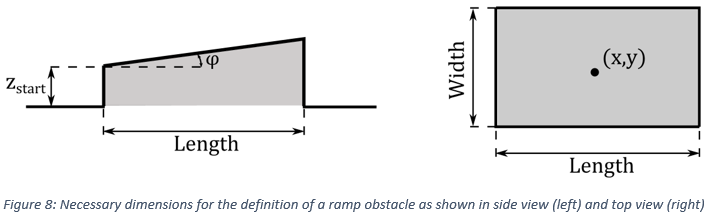Parameter Description Units
X X coordinate of obstacle’s center Length
Y Y coordinate of obstacle’s center Length
Z_START Z coordinate of obstacle's starting point Length
LENGTH Length of obstacle Length
WIDTH Width of obstacle Length
THETA Theta (orientation) angle of obstacle Angle
PHI Phi (inclination) angle of obstacle Angle
UMIN Parameter for tire-rigid road interaction (Fiala tire model) -
UMAX Parameter for tire-rigid road interaction (Fiala tire model) -
CSLIP Parameter for tire-rigid road interaction (Fiala tire model) Force
CALPHA Parameter for tire-rigid road interaction (Fiala tire model) Force
MAT Obstacle’s material. Optional parameter, default value is ‘SOFT_SOIL’ -

## Roof Obstacle

To create a roof obstacle, a new block (ROOF_OBSTACLE block) is necessary in the road data (.rdf) file that contains the following parameters: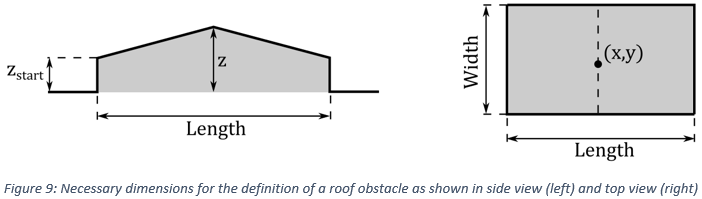Parameter Description Units
X X coordinate of obstacle’s center Length
Y Y coordinate of obstacle’s center Length
Z_START Z coordinate of obstacle's starting point Length
Z Z coordinate of obstacle's midpoint Length
LENGTH Length of obstacle Length
WIDTH Width of obstacle Length
THETA Theta (orientation) angle of obstacle Angle
UMIN Parameter for tire-rigid road interaction (Fiala tire model) -
UMAX Parameter for tire-rigid road interaction (Fiala tire model) -
CSLIP Parameter for tire-rigid road interaction (Fiala tire model) Force
CALPHA Parameter for tire-rigid road interaction (Fiala tire model) Force
MAT Obstacle’s material. Optional parameter, default value is ‘SOFT_SOIL’ -

## Sine Obstacle

To create a sine obstacle, a new block (SINE_OBSTACLE block) is necessary in the road data (.rdf) file that contains the following parameters: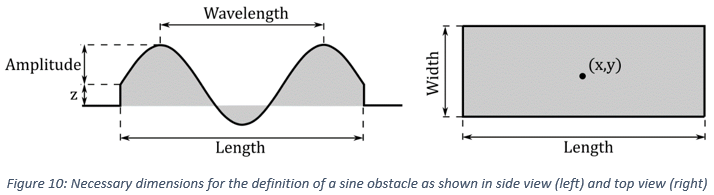Parameter Description Units
X X coordinate of obstacle’s center Length
Y Y coordinate of obstacle’s center Length
Z Z coordinate of obstacle Length
LENGTH Length of obstacle Length
WIDTH Width of obstacle Length
THETA Theta (orientation) angle of obstacle Angle
AMPLITUDE Amplitude of sine wave Length
WAVELENGTH Wavelength of sine wave Length
UMIN Parameter for tire-rigid road interaction (Fiala tire model) -
UMAX Parameter for tire-rigid road interaction (Fiala tire model) -
CSLIP Parameter for tire-rigid road interaction (Fiala tire model) Force
CALPHA Parameter for tire-rigid road interaction (Fiala tire model) Force
MAT Obstacle’s material. Optional parameter, default value is ‘SOFT_SOIL’ -

## Sine-sweep Obstacle

In this obstacle type, the amplitude and the frequency of the sine wave vary as a function of the longitudinal distance s. More specifically, two cases can be distinguished for the calculation of the obstacle’s height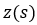.

### Linear Sweep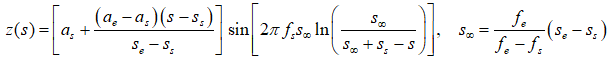### Logarithmic Sweep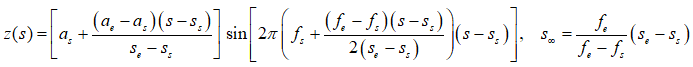To create a sine-sweep obstacle, a new block (SINE_SWEEP_OBSTACLE block) is necessary in the road data (.rdf) file that contains the following parameters:

Parameter Description Units
X X coordinate of obstacle’s center Length
Y Y coordinate of obstacle’s center Length
Z Z coordinate of obstacle Length
LENGTH Length of obstacle Length
WIDTH Width of obstacle Length
THETA Theta (orientation) angle of obstacle Angle
AMPLITUDE_START Amplitude of obstacle's starting point Length
WAVELENGTH_START Wavelength of obstacle's starting point Length
AMPLITUDE_END Amplitude of obstacle's end point Length
WAVELENGTH_END Wavelength of obstacle's end point Length
UMIN Parameter for tire-rigid road interaction (Fiala tire model) -
UMAX Parameter for tire-rigid road interaction (Fiala tire model) -
CSLIP Parameter for tire-rigid road interaction (Fiala tire model) Force
CALPHA Parameter for tire-rigid road interaction (Fiala tire model) Force
SWEEP_TYPE Sweep type (‘LINEAR’ or ‘LOGARITHMIC’). Optional parameter, default value is ‘LINEAR’ -
MAT Obstacle’s material. Optional parameter, default value is ‘SOFT_SOIL’ -

## Plank Obstacle

Using a plank obstacle, two different cases can be distinguished regarding the shape of its edges. In detail, beveled edges are used when the BEVEL_EDGE_LENGTH parameter is positive. On the other hand, round edges are created with a negative value of this parameter, where the radius of the corner is equal to |BEVEL_EDGE_LENGTH|.

To create a plank obstacle, a new block (PLANK_OBSTACLE block) is necessary in the road data (.rdf) file that contains the following parameters: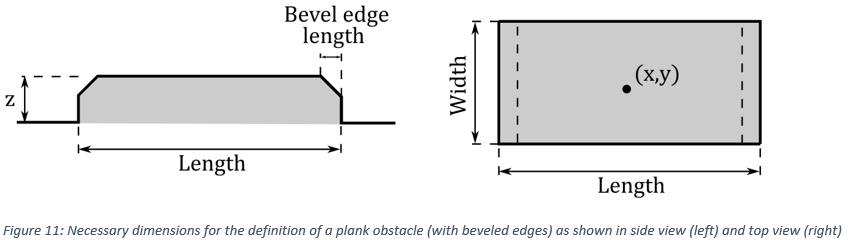Parameter Description Units
X X coordinate of obstacle’s center Length
Y Y coordinate of obstacle’s center Length
Z Z coordinate of obstacle Length
LENGTH Length of obstacle Length
WIDTH Width of obstacle Length
THETA Theta (orientation) angle of obstacle Angle
BEVEL_EDGE_LENGTH Length of the beveled edge (45° angle). If this parameter is negative, the obstacle is created with round edges, where the radius is equal to |BEVEL_EDGE_LENGTH| Length
UMIN Parameter for tire-rigid road interaction (Fiala tire model) -
UMAX Parameter for tire-rigid road interaction (Fiala tire model) -
CSLIP Parameter for tire-rigid road interaction (Fiala tire model) Force
CALPHA Parameter for tire-rigid road interaction (Fiala tire model) Force
MAT Obstacle’s material. Optional parameter, default value is ‘SOFT_SOIL’ -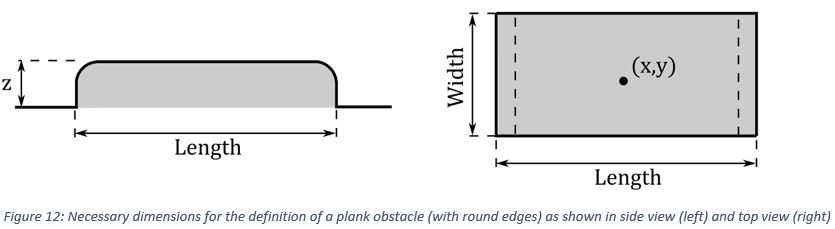## Custom Obstacle

The custom obstacle allows the creation of an arbitrary shape obstacle in the soft-soil road. The obstacle is defined as a Point Cloud Data (PCD) road added in the CUSTOM_OBSTACLE block.

The PCD are roads represented by Finite Element surfaces made of triangular mesh. The custom obstacle can be specified as a PCD by adding the nodes and elements table in the .rdf file or specifying an OptiStruct .fem file. For more information consult PCD [Point Cloud Data].

Parameter Description Units
X X coordinate of obstacle’s center Length
Y Y coordinate of obstacle’s center Length
Z Z coordinate of obstacle Length
THETA Theta (orientation) angle of obstacle Angle
UMIN Parameter for tire-rigid road interaction (Fiala tire model) -
UMAX Parameter for tire-rigid road interaction (Fiala tire model) -
CSLIP Parameter for tire-rigid road interaction (Fiala tire model) Force
CALPHA Parameter for tire-rigid road interaction (Fiala tire model) Force
FILE_PATH Point Could Data road file (.rdf) representing the obstacle. -
MAT Obstacle’s material. Optional parameter, default value is ‘SOFT_SOIL’ -

## OBSTACLES Block

Finally, in order to support more than one obstacle of each type (for example rectangular, circular, and so on), an extra block (OBSTACLES block) can be defined that contains a list of the obstacle names for each type, as shown in Fig. 14. It should be emphasized that this block is necessary only in cases where more than one obstacle of a specific type (for example rectangular, circular, and so on) exists in the model and only for the specific obstacle type. In all other cases, the obstacles are created using the blocks presented previously (RECT_OBSTACLE block for a rectangular obstacle, CIRC_OBSTACLE block for a circular obstacle, and so on). In addition, it should be noted that the obstacle names in each list need to be provided as comma-separated values, as depicted in the example below.

$-----------------------------------------------------OBSTACLES [OBSTACLES] RECT_OBSTACLE = 'RECT_OBS1,RECT_OBS2,RECT_OBS3' CIRC_OBSTACLE = 'CIRC_OBS1,CIRC_OBS2,CIRC_OBS3' BUMP_OBSTACLE = 'BUMP_OBS1,BUMP_OBS2,BUMP_OBS3' ROOF_OBSTACLE = 'ROOF_OBS1,ROOF_OBS2,ROOF_OBS3' RAMP_OBSTACLE = 'RAMP_OBS1,RAMP_OBS2,RAMP_OBS3' SINE_OBSTACLE = 'SINE_OBS1,SINE_OBS2,SINE_OBS3' SINE_SWEEP_OBSTACLE = 'SINE_SWEEP_OBS1,SINE_SWEEP_OBS2,SINE_SWEEP_OBS3' PLANK_OBSTACLE = 'PLANK_OBS1,PLANK_OBS2,PLANK_OBS3'$--------------------------------------------------------------RECTANGULAR_OBSTACLE
[RECT_OBS1]
X                      = 0.0
Y                      = 0.0
Z                      = 0.0
LENGTH                 = 1.0
WIDTH                  = 0.5
THETA                  = 0.0
UMIN                   = 0.8
UMAX                   = 1.0
CSLIP                  = 20000.0
CALPHA                 = 44000.0  

Figure 14: New block (OBSTACLES block) in order to support more than one obstacle of each type

## Property File Examples

### Rectangular Obstacle

$-----------------------------------------------------RECTANGULAR_OBSTACLE [RECT_OBSTACLE] X = 0.0$ X coordinate of obstacle's center
Y              = 0.0        $Y coordinate of obstacle's center Z = 0.0$ Z coordinate of obstacle
LENGTH         = 1.0        $Length of obstacle WIDTH = 0.5$ Width of obstacle
THETA          = 0.0        $Theta (orientation) angle of obstacle UMIN = 0.8$ Parameter for tire-rigid road interaction (Fiala tire model)
UMAX           = 1.0        $Parameter for tire-rigid road interaction (Fiala tire model) CSLIP = 20000.0$ Parameter for tire-rigid road interaction (Fiala tire model)
CALPHA         = 44000.0    $Parameter for tire-rigid road interaction (Fiala tire model) Figure 15: Example of deformable rectangular obstacle in road data (.rdf) file ### Circular Obstacle $-----------------------------------------------------CIRCULAR_OBSTACLE
[CIRC_OBSTACLE]
X              = 0.0        $X coordinate of obstacle's center Y = 0.0$ Y coordinate of obstacle's center
Z              = 0.0        $Z coordinate of obstacle RADIUS = 0.3$ Radius of circular obstacle
UMIN           = 0.8        $Parameter for tire-rigid road interaction (Fiala tire model) UMAX = 1.0$ Parameter for tire-rigid road interaction (Fiala tire model)
CSLIP          = 20000.0    $Parameter for tire-rigid road interaction (Fiala tire model) CALPHA = 44000.0$ Parameter for tire-rigid road interaction (Fiala tire model)

Figure 16: Example of deformable circular obstacle in road data (.rdf) file

### Bump Obstacle

$-----------------------------------------------------BUMP_OBSTACLE [BUMP_OBSTACLE] X = 0.0$ X coordinate of obstacle's center
Y              = 0.0        $Y coordinate of obstacle's center Z = 0.0$ Z coordinate of bump's center
WIDTH          = 0.3        $Width of obstacle RADIUS = 0.1$ Radius of bump
THETA          = 0.0        $Theta (orientation) angle of obstacle UMIN = 0.8$ Parameter for tire-rigid road interaction (Fiala tire model)
UMAX           = 1.0        $Parameter for tire-rigid road interaction (Fiala tire model) CSLIP = 20000.0$ Parameter for tire-rigid road interaction (Fiala tire model)
CALPHA         = 44000.0    $Parameter for tire-rigid road interaction (Fiala tire model) Figure 17: Example of deformable bump obstacle in road data (.rdf) file ### Ramp Obstacle $-----------------------------------------------------RAMP_OBSTACLE
[RAMP_OBSTACLE]
X              = 0.0        $X coordinate of obstacle's center Y = 0.0$ Y coordinate of obstacle's center
Z_START        = 0.0        $Z coordinate of obstacle's starting point LENGTH = 1.0$ Length of obstacle
WIDTH          = 0.5        $Width of obstacle THETA = 0.0$ Theta (orientation) angle of obstacle
PHI            = 0.087      $Phi (inclination) angle of obstacle UMIN = 0.8$ Parameter for tire-rigid road interaction (Fiala tire model)
UMAX           = 1.0        $Parameter for tire-rigid road interaction (Fiala tire model) CSLIP = 20000.0$ Parameter for tire-rigid road interaction (Fiala tire model)
CALPHA         = 44000.0    $Parameter for tire-rigid road interaction (Fiala tire model) Figure 18: Example of deformable ramp obstacle in road data (.rdf) file ### Roof Obstacle $-----------------------------------------------------ROOF_OBSTACLE
[ROOF_OBSTACLE]
X              = 0.0        $X coordinate of obstacle's center Y = 0.0$ Y coordinate of obstacle's center
Z_START        = 0.0        $Z coordinate of obstacle's starting point Z = 0.05$ Z coordinate of obstacle's midpoint
LENGTH         = 1.0        $Length of obstacle WIDTH = 0.5$ Width of obstacle
THETA          = 0.0        $Theta (orientation) angle of obstacle UMIN = 0.8$ Parameter for tire-rigid road interaction (Fiala tire model)
UMAX           = 1.0        $Parameter for tire-rigid road interaction (Fiala tire model) CSLIP = 20000.0$ Parameter for tire-rigid road interaction (Fiala tire model)
CALPHA         = 44000.0    $Parameter for tire-rigid road interaction (Fiala tire model) Figure 19: Example of deformable roof obstacle in road data (.rdf) file ### Sine Obstacle $-----------------------------------------------------SINE_OBSTACLE
[SINE_OBSTACLE]
X              = 0.0        $X coordinate of obstacle's center Y = 0.0$ Y coordinate of obstacle's center
Z              = 0.0        $Z coordinate of obstacle LENGTH = 1.0$ Length of obstacle
WIDTH          = 0.5        $Width of obstacle THETA = 0.0$ Theta (orientation) angle of obstacle
AMPLITUDE      = 0.05       $Amplitude of sine wave WAVELENGTH = 1.0$ Wavelength of sine wave
UMIN           = 0.8        $Parameter for tire-rigid road interaction (Fiala tire model) UMAX = 1.0$ Parameter for tire-rigid road interaction (Fiala tire model)
CSLIP          = 20000.0    $Parameter for tire-rigid road interaction (Fiala tire model) CALPHA = 44000.0$ Parameter for tire-rigid road interaction (Fiala tire model)

Figure 20: Example of deformable sine obstacle in road data (.rdf) file

### Sine-sweep Obstacle

$-----------------------------------------------------SINE_SWEEP__OBSTACLE [SINE_SWEEP_OBSTACLE] X = 0.0$ X coordinate of obstacle's center
Y                 = 0.0             $Y coordinate of obstacle's center Z = 0.0$ Z coordinate of obstacle
LENGTH            = 6.0             $Length of obstacle WIDTH = 0.3$ Width of obstacle
THETA             = 0.0             $Theta (orientation) angle of obstacle AMPLITUDE_START = 0.05$ Amplitude of obstacle's starting point
WAVELENGTH_START  = 1.0             $Wavelength of obstacle's starting point AMPLITUDE_END = 0.01$ Amplitude of obstacle's endpoint
WAVELENGTH_END    = 0.5             $Wavelength of obstacle's endpoint SWEEP_TYPE = 'LOGARITHMIC'$ Sweep type ('LINEAR' or 'LOGARITHMIC'). Default value is 'LINEAR'
UMIN              = 0.8             $Parameter for tire-rigid road interaction (Fiala tire model) UMAX = 1.0$ Parameter for tire-rigid road interaction (Fiala tire model)
CSLIP             = 20000.0         $Parameter for tire-rigid road interaction (Fiala tire model) CALPHA = 44000.0$ Parameter for tire-rigid road interaction (Fiala tire model)

Figure 21: Example of deformable sine-sweep obstacle in road data (.rdf) file

### Plank Obstacle

$-----------------------------------------------------PLANK_OBSTACLE [PLANK_OBSTACLE] X = 0.0$ X coordinate of obstacle's center
Y                   = 0.0         $Y coordinate of obstacle's center Z = 0.05$ Z coordinate of obstacle
LENGTH              = 1.0         $Length of obstacle WIDTH = 0.5$ Width of obstacle
THETA               = 0.0         $Theta (orientation) angle of obstacle BEVEL_EDGE_LENGTH = 0.02$ Length of the beveled edge
UMIN                = 0.8         $Parameter for tire-rigid road interaction (Fiala tire model) UMAX = 1.0$ Parameter for tire-rigid road interaction (Fiala tire model)
CSLIP               = 20000.0     $Parameter for tire-rigid road interaction (Fiala tire model) CALPHA = 44000.0$ Parameter for tire-rigid road interaction (Fiala tire model)

Figure 22: Example of deformable plank obstacle in road data (.rdf) file

### Custom Obstacle

$----------------------------------------------------------------CUSTOM_OBSTACLE [CUSTOM_OBS] X = -5.0$ X coordinate of obstacle's center
Y                   = 0.0                         $Y coordinate of obstacle's center Z = 0.0$ Z coordinate of obstacle
THETA               = 0.0                         $Theta (orientation) angle of obstacle UMIN = 0.8$ Parameter for tire-rigid road interaction (Fiala tire model)
UMAX                = 1.0                         $Parameter for tire-rigid road interaction (Fiala tire model) CSLIP = 20000.0$ Parameter for tire-rigid road interaction (Fiala tire model)
CALPHA              = 44000.0                     $Parameter for tire-rigid road interaction (Fiala tire model) FILE_PATH = 'PCD_road_obstacle.rdf'$ Point Could Data road file representing the obstacle

Figure 23: Example of deformable custom obstacle in road data (.rdf) file